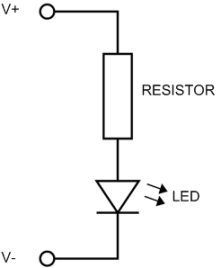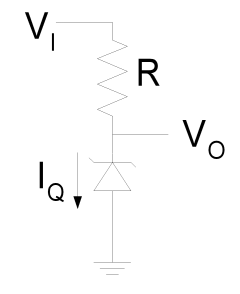# CalculatorsVoltage (V) Current (I) Ohms (R) Power (W)Supply Voltage Volts Diode Forward Voltage Volts Diode Current milliamps (mA) Calculated Resistance Ohms Next Standard Resistor Ohms Resistor Power milliwatts (mW)Vi: Enter source voltage (must be at least 2V higher than desired output.) Vo: Enter desired output voltage (zener voltage) Iq: Enter load current in mA. Add 10% to be on safe side. Prefered Zener value: V Zener diode power rating: mW Select a zener diode with a higher rating than the value above Resistor value: Ohms Prefered Resistor value: Ohms Select a resistor with a value as close as possible to the value above a little below is better than a little above. Resistor power rating: mW Select a resistor with a power rating higher than the value above.
Powered By Elbow Grease